• 一维Burgers方程 一维burgers方程为： 由于等式右边可以进行积分： 利用F = u**2，则方程为： 假设u初始为阶跃函数： 数值解法采用MacCormack格式： 但是这一解法，有失真的性质，后面具体介绍。 所以根据...
一维Burgers方程
一维burgers方程为：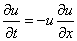由于等式右边可以进行积分：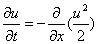利用F = u**2，则方程为：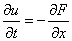假设u初始为阶跃函数：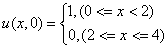数值解法采用MacCormack格式：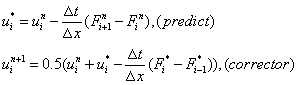但是这一解法，有失真的性质，后面具体介绍。
所以根据这一格式，可以直接数值求解，并利用matplotlib画出动态的数值图形，具体代码如下：
# -*- coding: utf-8 -*-
"""
Created on Tue Jan 20 14:32:23 2015
1D burges equation
@author: myjiayan
"""

import numpy as np
import matplotlib.pyplot as plt
from matplotlib import animation

def u_initial():
first = np.ones(40)
second = np.zeros(41)
result = np.array(list(first)+list(second))
return result

def computeF(u):
F = 0.5*u**2
return F

def maccormack(u,nt,dt,dx):
un = np.zeros((nt,len(u)))
un = u.copy()
ustar = u.copy()

for t in xrange(1,nt):
F = computeF(u)
ustar[:-1] = u[:-1] - (F[1:]-F[:-1])*dt/dx
ustar[-1] = 0.0
Fstar = computeF(ustar)
un[t,1:] = 0.5*(u[1:]+ustar[1:]-(Fstar[1:]-Fstar[:-1])*dt/dx)
un[t,0] = 1.0
u = un[t].copy()

return un

if __name__ == '__main__':
nx = 81
nt = 70
dx = 4.0/(nx-1)

def animate(data):
x = np.linspace(0,4,nx)
y = data
line.set_data(x,y)
return line,

u = u_initial()
sigma = .5
dt = sigma*dx

un = maccormack(u,nt,dt,dx)
fig = plt.figure();
ax = plt.axes(xlim=(0,4),ylim=(-.5,2));
line, = ax.plot([],[],lw=2);
anim = animation.FuncAnimation(fig, animate, frames=un, interval=50)
plt.show()
直接将代码保存为burgers.py文件，打开terminal：
\$python burgers.py
数值结果就以动态的形式表现出来了。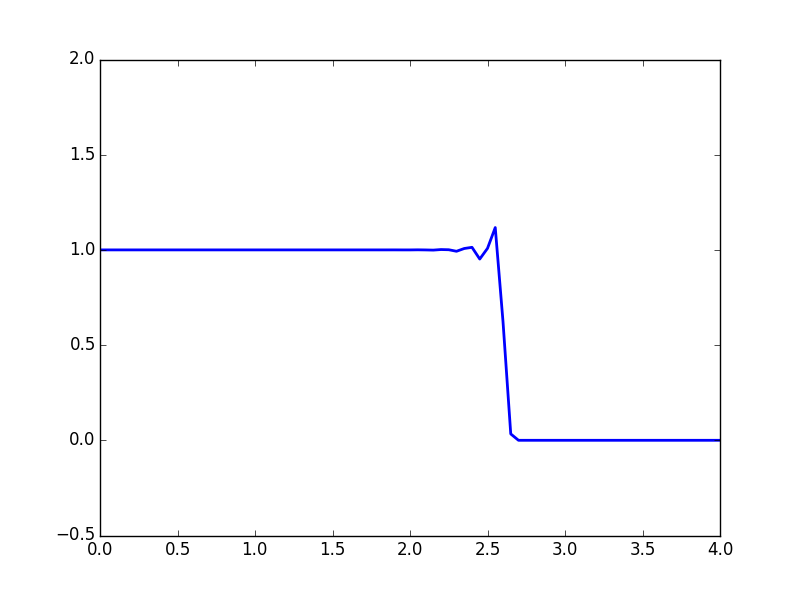很明显，数值结果失真了，数值结果中u的值竟然比1大，如何改进MacCormack格式呢？
我们在预测步上加一个人工项，新的格式为：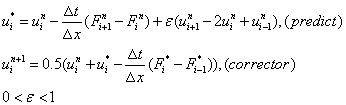选取Ephsilon为0.5后改进格式得到的数值结果为：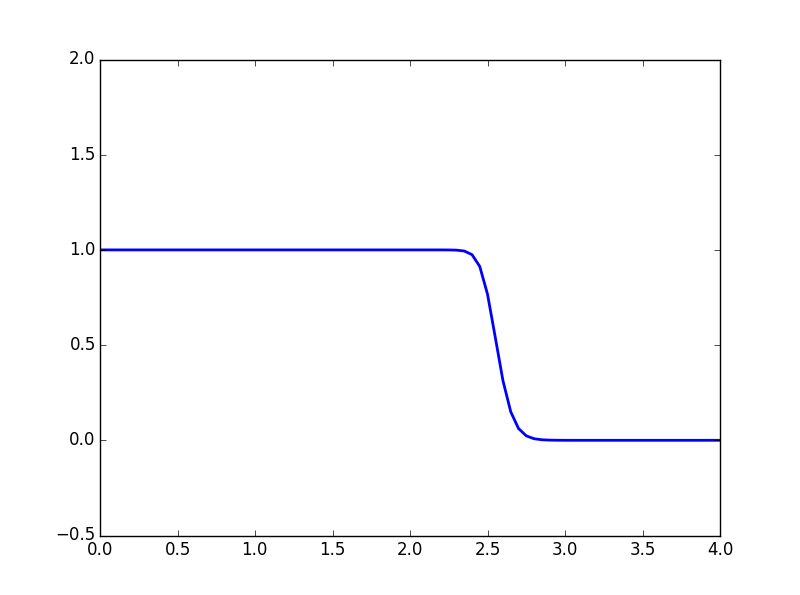比1大的值已经消失。


展开全文python 数值方法 animation
• 学过计算流体力学的朋友，在控制方程数学性质方面，可能会遇到控制方程类型证明问题，例如：一维热传导方程、拉普拉斯方程一维波动方程推导，建议多从克莱默法则入手，构造方程组，求解特征线。 ...
学过计算流体力学的朋友，在控制方程数学性质方面，可能会遇到控制方程类型证明问题，例如：一维热传导方程、拉普拉斯方程、一维波动方程推导，建议多从克莱默法则入手，构造方程组，求解特征线。二阶的导数部分，采用一阶导数替换，令,再结合全微分方程即可。

展开全文• 一维对流扩散方程的学习——来自流沙公众号 认识： 1、时间步长越小越靠后移动；网格越小则波的形状越一致,波形失真在减小，引出Courant数：u*...4、明白了一维方程编程的简单运算，基础 import numpy as np # n...
一维常系数对流方程的学习——来自流沙公众号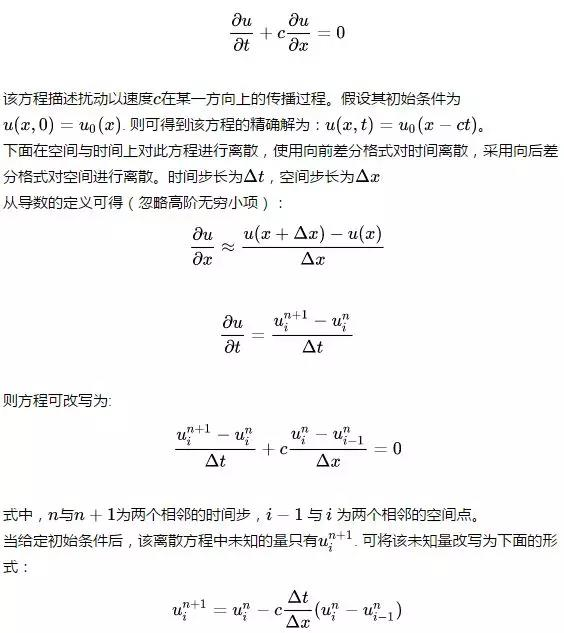认识：
1、时间步长越小越靠后移动；网格越小则波的形状越一致,波形失真在减小，引出Courant数：u*dt/dx<sigma；
2、对numpy中的ones（）、linspace（）、zeros（）有基础认识；
3、再次练习了matplotlib中的pyplot。
4、明白了一维方程编程的简单运算，基础
import numpy as np
# numpy是Python的一个科学计算的库，提供了矩阵运算的功能，其一般与Scipy、matplotlib一起使用。
import matplotlib.pyplot as plt

def linearconv(nx):
dx = 2/(nx - 1) # 空间网格步长，x方向总长度为2
nt = 25 # 总的时间步长
sigma = 0.8  # Courant数
c = 1 # 常数
dt =  sigma * dx / c  # 时间步长

# 指定初始条件
u = np.ones(nx)
u[int(0.5/dx):int(1/dx + 1)] = 2 # 这一行实际上是制造了一个方波(0.5--1)
# 下面将初始条件画出来
plt.plot(np.linspace(0,2,nx),u,'r',linewidth=3,label = 'init') # linspace用于产生x1,x2之间的N点行矢量,其中x1、x2、N分别为起始值、终止值、元素个数

# 计算25个时间步后的波长
un = np.ones(nx)
for n in range(nt):
un = u.copy() # 后面的计算会改变u，故将u拷贝到un
for i in range(1,nx):
u[i] = un[i] - c * dt / dx * (un[i] - un[i-1])

plt.plot(np.linspace(0,2,nx),u,'b',linewidth = 3, label = 'current')
plt.xlabel("distance")
plt.ylabel("speed")
plt.legend()
plt.show()

linearconv(10001)
# 时间步长越小越靠后移动；网格越小则波的形状越一致,波形失真在减小，故引出了Courant数：u*dt/dx<sigma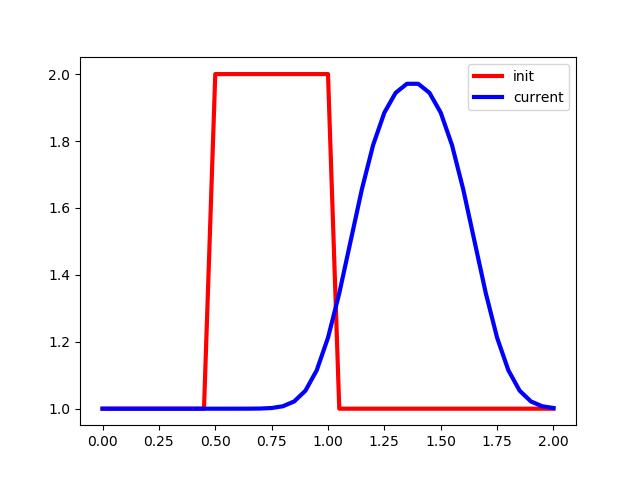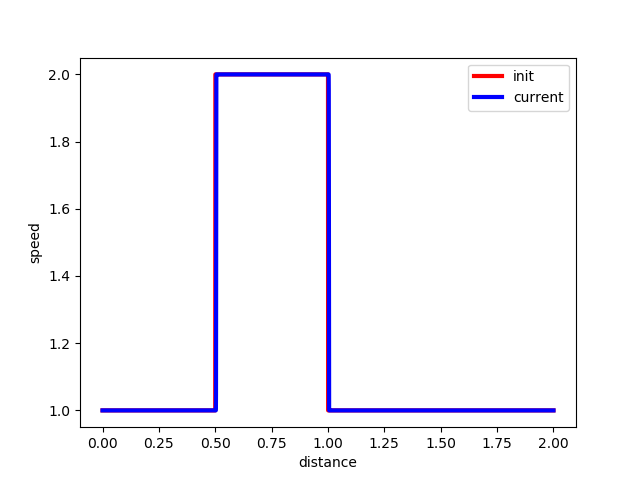展开全文• 数值计算——一维非线性方程求解 1、二分法  把函数f(x)的零点所在的区间[a，b](满足f(a）●f(b） package com.kexin.lab6; import java.text.DecimalFormat; /** * 二分法求解非线性方程组 * @author ...
数值计算——一维非线性方程求解
1、二分法
把函数f(x)的零点所在的区间[a，b](满足f(a）●f(b）<0)“一分为二”，得到[a，m]和[m，b]。根据“f(a)●f(m)<0”是否成立，取出零点所在的区间[a，m]或[m，b]，仍记为[a，b]。所对得的区间[a，b]重复上述步骤，直到包含零点的区间[a，b]“足够小”，则[a，b]内的数可以作为方程的近似解。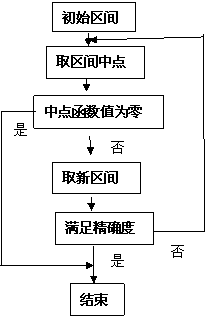package com.kexin.lab6;

import java.text.DecimalFormat;

/**
* 二分法求解非线性方程组
* @author KeXin
*
*/
public class Bisection {
//精度
final static double tol = 0.000001;
/**
* 定义函数x^3-2x-5，返回指定x的函数值
* @param a
* @return
*/
public static double Function(double a){
//		double result = Math.pow(a, 2)-4*Math.sin(a);	//书上的例题测试
double result = Math.pow(a, 3)-2*a-5;
return result;
}
/**
* 二分法定有根区间
* @param a
* @param b
* @return
*/
public static void Bisect(double a,double b){
DecimalFormat df  = new DecimalFormat("#.000000");
double m;
System.out.println("a\t\tF(a)\t\tb\t\tF(b)");
while((b-a)>tol){
m = a+(b-a)/2;
if(Math.signum(Function(a))==Math.signum(Function(m))){
a = m;
}else{
b = m;
}
System.out.println(df.format(a)+"\t"+df.format(Function(a))+"\t"+df.format((b))+"\t"+df.format(Function(b)));
}
}
public static void main(String[] args) {
double a = 1;
double b = 3;
Bisect(a,b);
}

}
2、牛顿法
在非线性方程ƒ(x)＝0的零点x＝x邻域内，函数 ƒ(x)连续可微且ƒ┡(x)不为零,xn(n=0,1,2,…)是x的近似值,则在此邻域，用线性函数g(x)
= f+f'(x-x0)代替f(x)并将g(x)的零点作为新的x近似值进行迭代求解：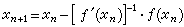牛顿法收敛很快，而且可求复根，缺点是对重根收敛较慢，但是要求函数的一阶导数存在。
package com.kexin.lab6;

import java.text.DecimalFormat;

/**
* 牛顿法求非线性方程组
*
* @author KeXin
*
*/
public class Newton {
// 精度
final static double tol = 0.000001;

/**
* 定义函数x^3-2x-5，返回指定x的函数值
*
* @param a
* @return
*/
public static double Function(double a) {
// double result = Math.pow(a, 2)-4*Math.sin(a); //书上的例题测试
double result = Math.pow(a, 3) - 2 * a - 5;
return result;
}

/**
* 定义函数x^2-2即F的导数，返回指定x的函数值
*
* @param a
* @return
*/
public static double Function1(double a) {
// double result = 2*a-4*Math.cos(a); //书上的例题测试
double result = 3 * Math.pow(a, 2) - 2;
return result;
}
/**
* 牛顿法
*
* @param a
* @param b
* @return
*/
public static void NewtonF(double x, int k) {
DecimalFormat df = new DecimalFormat("#.000000");
System.out.println("k\tx\t\tF(x)\t\tF1(x)\t\tH(x)");
double f, f1, f2;
while (k >= 0) {
f = Function(x);
f1 = Function1(x);
f2 = -f/f1;
System.out.println(k + "\t" + df.format(x) + "\t" + df.format(f) + "\t" + df.format(f1) + "\t" + df.format(f2));
if ((Math.abs(f) < tol && (Math.abs(f2) / Math.abs(x)) < tol))
break;
x = x + f2;
k--;
}
}

public static void main(String[] args) {
int k = 4;
double x = 3.0;
NewtonF(x, k);
}

}
3、割线法
用区间[tk-1，tk](或[tk，tk-1])上的割线近似代替目标函数的 导函数的曲线。并用割线与横轴交点的横坐标作为方程式的根的近似。
package com.kexin.lab6;

import java.text.DecimalFormat;

/**
* 割线法求解非线性方程
*
* @author KeXin
*
*/
public class Secant {
// 精度
final static double tol = 0.000001;

/**
* 定义函数x^3-2x-5，返回指定x的函数值
*
* @param a
* @return
*/
public static double Function(double a) {
//		 double result = Math.pow(a, 2)-4*Math.sin(a); //书上的例题测试
double result = Math.pow(a, 3) - 2 * a - 5;
return result;
}
/**
* 牛顿法
*
* @param a
* @param b
* @return
*/
public static void SecantF(double x0,double x, int k) {
DecimalFormat df = new DecimalFormat("#.000000");
System.out.println("k\tx\t\tF(x)\t\tH(x)");
double f, f1, h = 0,temp;
while (k >= 0) {
f = Function(x);
f1 = Function(x0);
System.out.println(k + "\t" + df.format(x) + "\t" + df.format(f) + "\t"+df.format(h));
temp = x;
x = x - f*(x-x0)/(f-f1);
x0 = temp;
h = x-x0;
k--;
}
}
public static void main(String[] args) {
SecantF(3,1,8);
}

}

展开全文二分法 割线法
• 通过消元法得到新的低阶方程组，递归求解新方程组，最后求解所消去变元。 class Program { static void Main(string[] args) { List list = new List(); decimal[] equation = new decim
• 摘要：采用FTCS显格式、Dufort-Frankel显格式、Lassonen隐格式、Grank-Nicolson隐格式对一维扩散方程进行数值计算，得到不同时间y方向扩散的速度。结果表明了不同差分格式的差别。 关键词：扩散方程；差分格式；...
• a为方程中的系数b，可任取 I = 1 / h ; N = maxt / deltat ; sol = zeros ( fix ( I + 1 ) , fix ( N + 1 ) ) ; mu = a * deltat / h ^ 2 ; vetx = [ 0 : h : 1 ] ; veti = ones ( I - 1 , ...matlab
• 伯格斯方程(Burgers equation) 是个模拟冲击波的传播和反射的非线性偏微分方程。 伯格斯方程也称为粘性伯格斯方程，只适用于一个空间维度。
• 热传导方程一维问题问题背景问题抽象离散方法1 向前差分迭代格式初值设定取参数C语言实现fortran实现画图 hexo博客为啥用不了了，将就着先用这个博客吧 一维问题 问题背景 摘抄自网络 ：在距离为L的两个半无限长壁...算法
• 2、第次接触matplotlib中ion()和ioff()。ion()开启了交互模式,ioff() 感觉像暂停图像 同时，查阅其他博客得到： python可视化库matplotlib有两种显示模式： 阻塞（block）模式 交互（interactive）模式 在...
• 一维泊松方程 二维泊松方程（图像处理）图像处理
• 对于变量ϕ\phiϕ的输运方程为： ∂(ρϕ)∂t+∇⋅(ρϕu)=∇⋅(Γ∇ϕ)+Sϕ(1) \frac{\partial ( \rho \phi)}{\partial t} + \nabla \cdot (\rho \phi \bold u) = \nabla \cdot (\Gamma \nabla \phi) + S_\phi \tag...
• 含matlab程序，个人感觉很有帮助，在研究传热学的可以下来看看matlab程序
• 某些二阶线性偏微分方程，可分解为两个一阶线性偏微分方程，有可能积分求出通解。例如，二阶方程 ∂2u∂x∂y+∂u∂x=0 \frac{\partial^2u}{\partial x\partial y}+\frac{\partial u}{\partial x}=0 ∂x∂y∂2u​+∂...
• 偏微分方程的数值解(二): 一维状态空间的偏微分方程的 MATLAB 解法 偏微分方程的数值解(三): 化工应用实例 ----------触煤反应装置内温度及转换率的分布 偏微分方程的数值解(四): 化工应用————扩散系统之浓度...
• 在有限元程序中，刚度方程[K]建立完毕，节点力向量F经过了非齐次边界条件处理、等效...迭代法则用于复杂的方程组，在精确解难以求出或求解成本太高时，通过次次迭代近似求解，逼近精确解，当达到可接受的精度时，...
• 请构造次有限元求解上述方程并给出相应误差估计; 请构造二次有限元求解上述方程并给出相应误差估计. 问题分析 取检验函数空间 V=H01(Ω),V=H_0^1(\Omega),V=H01​(Ω), 则对 ∀ v∈V,\forall\,v\in V,∀v∈V, 有...算法
• 主要需要的专业知识：计算热物理，matlab编程语言（研究生课程，还是需要一些基础知识的） 举个栗子： 对于具有常扩散系数的一维扩散方程： 其中D是常数，初始条件为： 边界条件为： 按照将方程和定解条件无量纲化，...算法 matlab
• 使用python语言和MATLAB求解二拉普拉斯方程实例 迭代
• 假设棒的截面内温度均匀，问题简化为一维导热模型，其控制方程为 ddx(kAdTdx)−hP(T−T∞)=0(1) \frac{d}{dx} \left( kA\frac{dT}{dx} \right) - hP(T-T_\infin)=0 \tag{1} dxd​(kAdxdT​)−hP(T−T∞​)=0(1) ...python
• 如何看待个n个未知量的n线性方程组？ 可以总结为下列的公式： AX ＝ b A是个nxn的矩阵，x和b是个列向量，未知量x ＝ { x1, x2, ..... xn}， b ＝ { b1, b2, ..... bn} 上面的公式可以从两个方面看： ...
• 使用有限差分的二波动方程数值解。 该代码通过有限差分法在正方形板上求解2D波动方程，并绘制2D运动和绝对误差的动画。为简单起见，所有单位均已标准化。它使用Courant-Friedrich-Levy稳定性条件。 ...
• 表达式 证明 特点 一般式 A1x... 参数方程 x=x0+mt y=y0+nt z=z0+pt 有直线(x-x0)/m=(y-y0)/n=(z-z0)/p，令t=(x-x0)/m=(y-y0)/n=(z-z0)/p，则有： x=x0+mt y=y0+nt z=z0+pt 1、方向向量是(m,n,p)； 2、过点(x0,y0,z0)。
• ## 空间三维直线方程求解方法

万次阅读 多人点赞 2018-01-17 10:22:48
2018-01-17 创建人：Ruo_Xiao 邮箱：xclsoftware@163.com 1、一般方程：两个相交的平面确定条直线。 2、点向式：点和直线方向可以确定条直线。 3、两点式：空间两个点确定条直线。
• Matlab偏微分方程快速上手：使用pdetool工具箱求解二偏微分方程，适用于数学建模、数学实验，简单的偏微分方程数值计算与工程问题。matlab 数学建模
• 最近在读《ocean modelling for beginners》这本书，对于做海洋... 第五章讲的是二浅水方程，书中进行了一些案例的模拟，并给出了相关代码。其中，模拟部分的代码使用Fortran，后处理部分使用Scilab。我试着用matl算法 matlab
• 方形板热方程的稳态差分有限差分法 该代码旨在解决2D板中的热方程。 使用固定的边界条件“狄利克雷条件”和所有节点的初始温度，可以解决直到达到稳态，并在代码中选择了公差值。 解决后，图形仿真显示为......